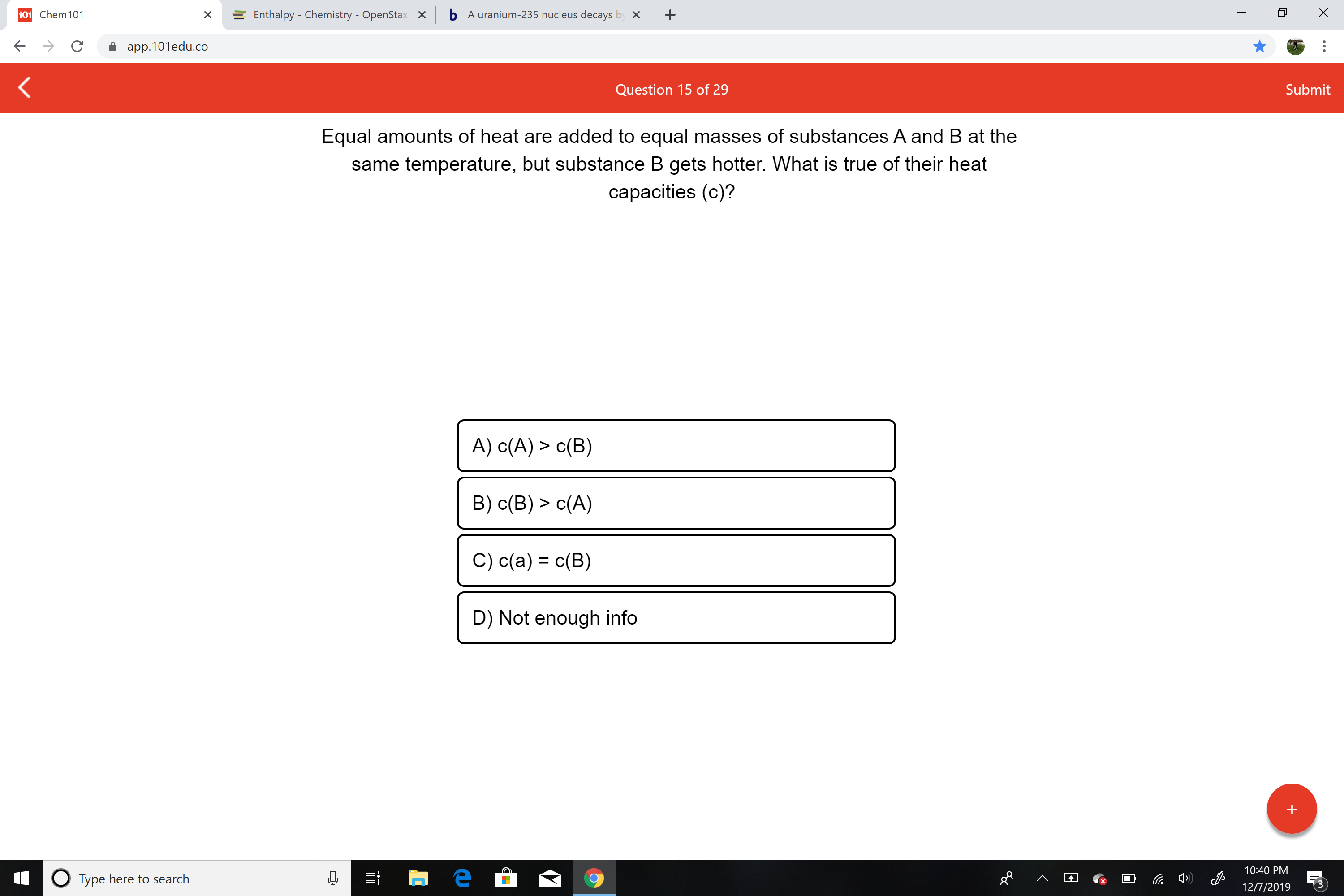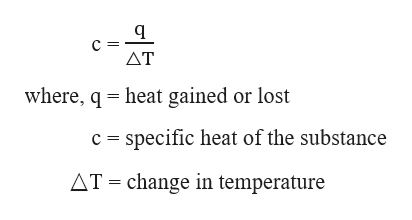# b A uranium-235 nucleus decays by x101 Chem101+Enthalpy - Chemistry - OpenStaxXapp.101edu.coSubmitQuestion 15 of 29Equal amounts of heat are added to equal masses of substances A and B at thesame temperature, but substance B gets hotter. What is true of their heatcapacities (c)?A) c(A) > c(B)B) c(B) > c(A)C) c(a) = c(B)D) Not enough info10:40 PMO Type here to search12/7/2019

Question
1 viewshelp_outlineImage Transcriptioncloseb A uranium-235 nucleus decays by x 101 Chem101 + Enthalpy - Chemistry - OpenStax X app.101edu.co Submit Question 15 of 29 Equal amounts of heat are added to equal masses of substances A and B at the same temperature, but substance B gets hotter. What is true of their heat capacities (c)? A) c(A) > c(B) B) c(B) > c(A) C) c(a) = c(B) D) Not enough info 10:40 PM O Type here to search 12/7/2019 fullscreen
check_circle

Step 1

The heat capacity, c of substance is given as :help_outlineImage TranscriptioncloseAT where, q = heat gained or lost c = specific heat of the substance AT = change in temperature fullscreen
Step 2

Given that, equal amounts of heat are added to equal masses of substances A and B at the same temperature.

But substance B gets hotter, which means that the final temperature of B is more than A, as such, the △T value of B will be more than A.

From the heat capacity formula, it is evident that heat capac...

### Want to see the full answer?

See Solution

#### Want to see this answer and more?

Solutions are written by subject experts who are available 24/7. Questions are typically answered within 1 hour.*

See Solution
*Response times may vary by subject and question.
Tagged in

### Chemical Thermodynamics